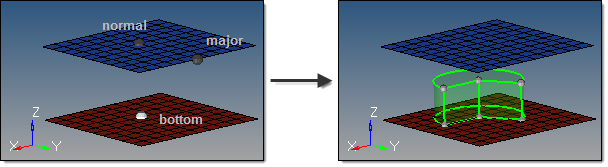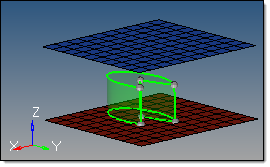﻿ cylinder partial subpanel (Solids panel)
Manufacturing Solutions

# cylinder partial subpanel (Solids panel)# cylinder partial subpanel (Solids panel)This subpanel creates three-dimensional, partial-cylinder solid primitives.Eight inputs are required to create a cylinder using this method:

 • The bottom center node defines the center of the bottom cylinder face.
 • The vector between the bottom center node and the normal vector node defines the cylinder axis, thereby indicating the cylinder orientation.  This does not indicate the actual cylinder height.
 • The vector between the bottom center node and the major vector node determines the zero-degree point of an arc defining the curved surface of the partial cylinder.  This arc extends in a direction based on the normal vector using the right-hand rule, with its start angle and end angle specified relative to this vector.
 • The base radius defines the radius of the top and bottom cylinder faces.
 • The height defines the cylinder height.
 • The start angle defines the starting arc angle, measured from the major vector node in a direction based on the normal vector using the right-hand rule.
 • The end angle defines the ending arc angle, measured from the major vector node in a direction based on the normal vector using the right-hand rule.  The difference between this and the start angle determines the arc of the partial cylinder, and therefore the arc of the cutout in the partial cylinder.  For example, if your start angle is 15 degrees, and your end angle is 285 degrees, the resulting cylinder has a base with a 270 degree arc and a 90 degree cut.
 • The axis ratio represents a percentage of the major vector.  This value must be greater than zero and less than or equal to 1.0.  Values other than 1.0 create oval-shaped cylinders instead of circular ones.With a ratio of 0.5 the cone is half as wide as its length.